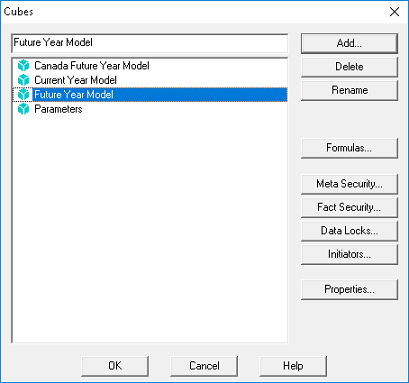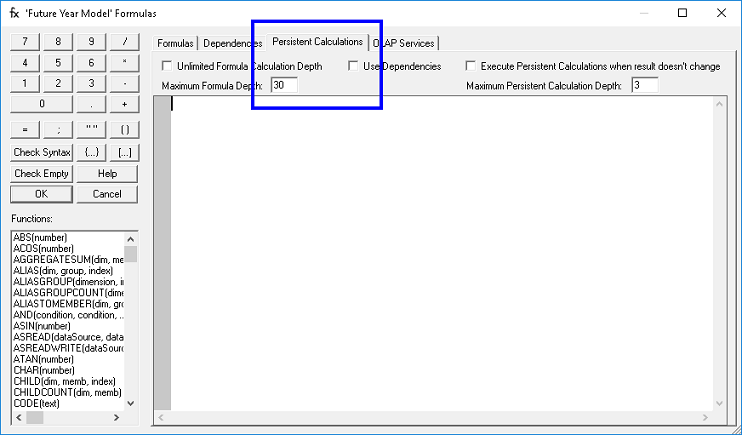Introduction to PowerOLAP’s Persistent CalculationsPersistent Calculations is a way to improve the performance of PowerOLAP models, particularly the performance of computing Cube formulas.

Persistent Calculations are computed at Data Entry time, instead of Slice calculation time (moving the calculation of formulas away from the actual slice calculation or MS Excel calculation time to the data entry time).

Results of the calculation are stored data directly to disk, values are written to disk as if they were fact data. The formula engine is not needed to recall those data points governed by Persistent Calculations, making these calculations very efficient and fast.

This is very useful for very large models with significant amounts of Dimensions and with large numbers of calculations on many detailed points.

When to Use Persistent Calculations

Persistent Calculations could be used in simple data transfer formulas that do not require computation every time a Slice is recalculated, such as those that move detail data from one Cube to another (it may balance the performance load in your Cube between what is calculating solely in RAM (formulas) and what has been written to disk and is less often recalculated).

Persistent Calculations must be based on Detail Members; At least one of the underlying driving members of the Persistent Calculation must be a Detail point.  This does not apply to Aggregate level or formula calculated values.

How to Use Persistent Calculations

Syntax

The syntax for the Persistent Calculations is the same with the syntax for the PowerOLAP formulas, thus:

<LHS> = <RHS> ;

Where:

 DESCRIPTION LHS is a Left Hand Side Range RHS is a Right Hand Side. ; semicolon

Accessing the Persistent Calculations Editor

The Persistent Calculations formula editor could be accessed from the Formula Editor of PowerOLAP®.

1.   In the PowerOLAP ribbon, select Model tab and Cubes command.
The Cubes dialog box is opened.

2.   Select a Cube (e.g., Future Year Model) and click the Formulas Button.
This will show the PowerOLAP Formula Editor window.3.   Click the Persistent Calculations tab.4.   Enter Persistent Calculations formula in the editor.